## Role of priors

Applying the updating reasoning to our box game, the Bayes factor of interest is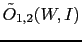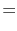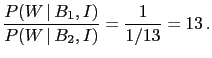(7)

As it was remarked, this number would give the required odds if the hypotheses were initially equally likely. But how strong are the initial relative beliefs on the two hypotheses? Unfortunately', we cannot perform a probabilistic inversion if we are unable to assign somehow prior probabilities to the hypotheses we are interested in.9Indeed, in the formulation of the problem I on purpose passed over the relevant pieces of information to evaluate the prior probabilities (it was said that there are two types of boxes'', not there are two boxes''!). If we specify that we had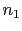boxes of type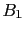andof the other kind, then the initial odds areand the final ones will be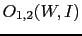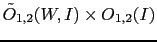(8)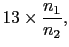(9)

from which we get (just requiring that the probability of the two hypotheses have to sum up to one10)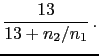(10)

If the two hypotheses were initially considered equally likely, then the evidencemakes13 times more believable than, i.e., or approximately 93%. On the other hand, ifwas a priori much less credible than, for example by a factor 13, just to play with round numbers, the same evidence madeandequally likely. Instead, if we were initially in strong favor of, considering it for instance 13 times more plausible than, that evidence turned this factor into 169, making us 99.4% confident - highly confident, some would even say practically sure'! - that the box is of type.

Giulio D'Agostini 2010-09-30